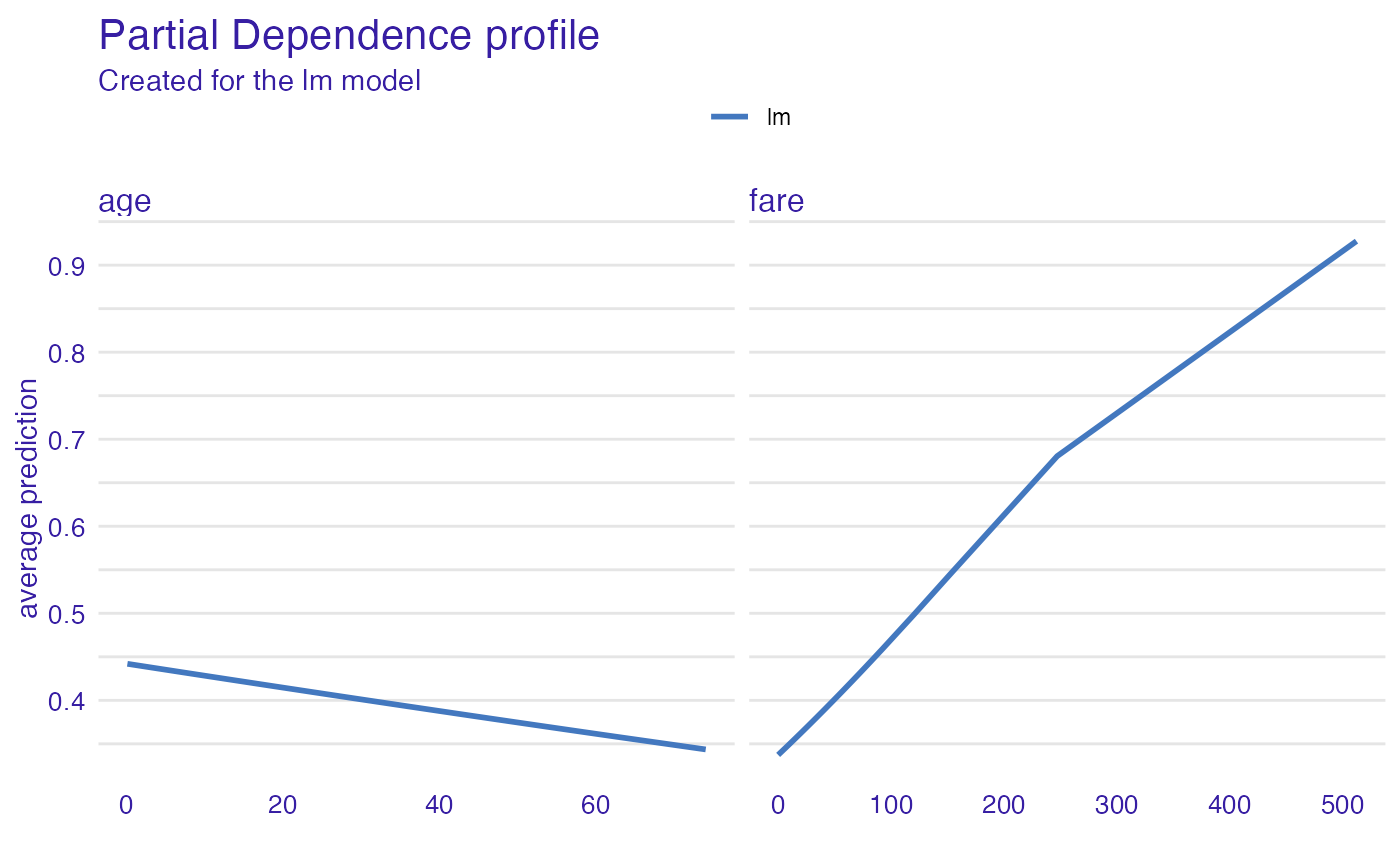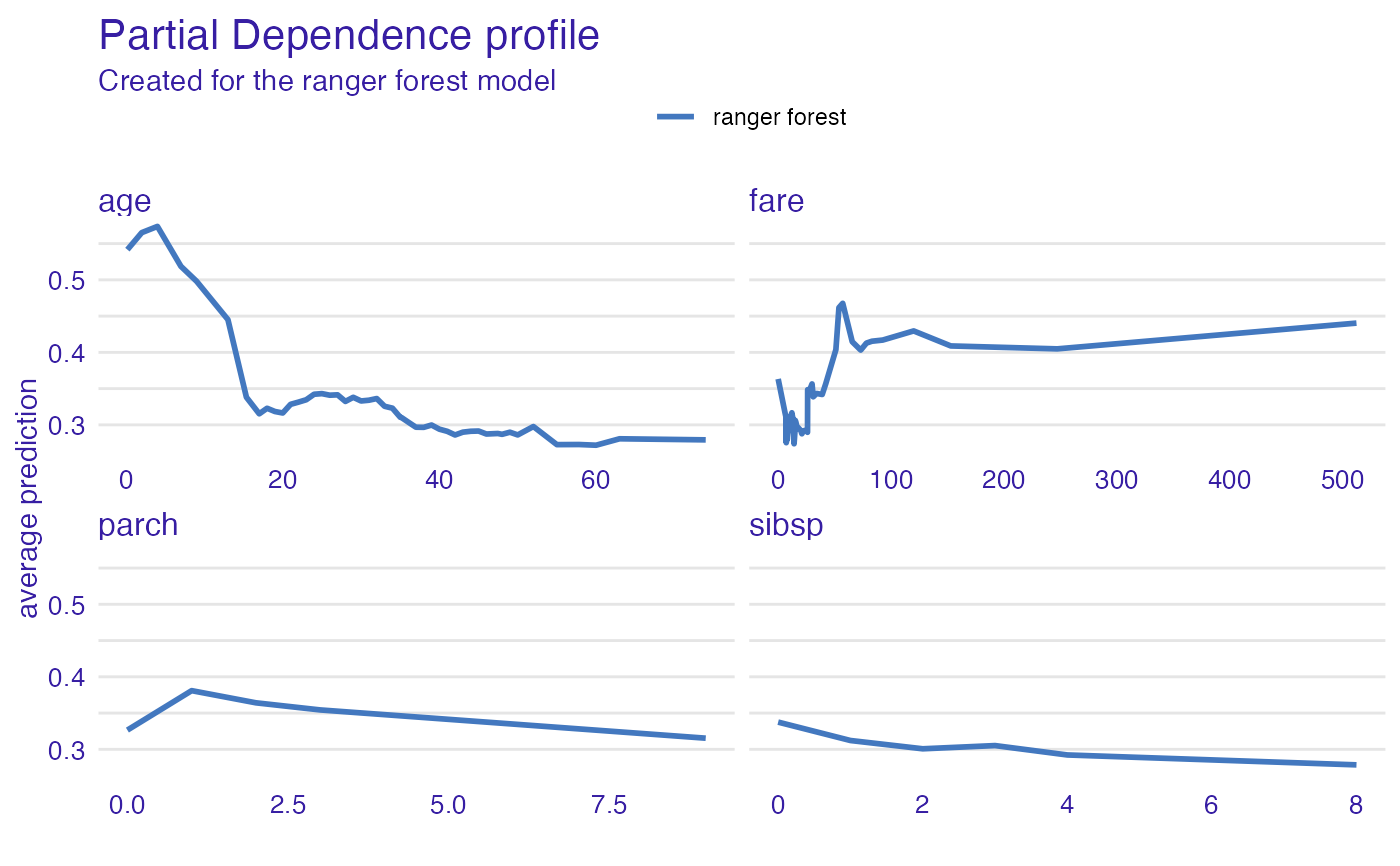Partial Dependence Profiles are averages from Ceteris Paribus Profiles. Function partial_dependence calls ceteris_paribus and then aggregate_profiles.

partial_dependence(x, ...)

# S3 method for explainer
partial_dependence(
x,
variables = NULL,
N = 500,
variable_splits = NULL,
grid_points = 101,
...,
variable_type = "numerical"
)

# S3 method for default
partial_dependence(
x,
data,
predict_function = predict,
label = class(x),
variables = NULL,
grid_points = 101,
variable_splits = NULL,
N = 500,
...,
variable_type = "numerical"
)

# S3 method for ceteris_paribus_explainer
partial_dependence(x, ..., variables = NULL)

partial_dependency(x, ...)

## Arguments

x

an explainer created with function DALEX::explain(), an object of the class ceteris_paribus_explainer or or a model to be explained.

...

other parameters

variables

names of variables for which profiles shall be calculated. Will be passed to calculate_variable_split. If NULL then all variables from the validation data will be used.

N

number of observations used for calculation of partial dependence profiles. By default 500.

variable_splits

named list of splits for variables, in most cases created with calculate_variable_split. If NULL then it will be calculated based on validation data avaliable in the explainer.

grid_points

number of points for profile. Will be passed to calculate_variable_split.

variable_type

a character. If "numerical" then only numerical variables will be calculated. If "categorical" then only categorical variables will be calculated.

data

validation dataset, will be extracted from x if it's an explainer NOTE: It is best when target variable is not present in the data

predict_function

predict function, will be extracted from x if it's an explainer

label

name of the model. By default it's extracted from the class attribute of the model

## Value

an object of the class aggregated_profiles_explainer

## Details

Find more details in the Partial Dependence Profiles Chapter.

## Examples

library("DALEX")

model_titanic_glm <- glm(survived ~ gender + age + fare,
data = titanic_imputed, family = "binomial")

explain_titanic_glm <- explain(model_titanic_glm,
data = titanic_imputed[,-8],
y = titanic_imputed[,8],
verbose = FALSE)

pdp_glm <- partial_dependence(explain_titanic_glm,
N = 25, variables = c("age", "fare"))
#> Top profiles    :
#>   _vname_ _label_       _x_    _yhat_ _ids_
#> 1    fare      lm 0.0000000 0.3373059     0
#> 2     age      lm 0.1666667 0.4419618     0
#> 3     age      lm 2.0000000 0.4394139     0
#> 4     age      lm 4.0000000 0.4366398     0
#> 5    fare      lm 6.1793080 0.3449402     0
#> 6     age      lm 7.0000000 0.4324893     0
plot(pdp_glm)# \donttest{
library("ranger")

model_titanic_rf <- ranger(survived ~., data = titanic_imputed, probability = TRUE)

explain_titanic_rf <- explain(model_titanic_rf,
data = titanic_imputed[,-8],
y = titanic_imputed[,8],
label = "ranger forest",
verbose = FALSE)

pdp_rf <- partial_dependence(explain_titanic_rf, variable_type = "numerical")
plot(pdp_rf)pdp_rf <- partial_dependence(explain_titanic_rf, variable_type = "categorical")
plotD3(pdp_rf, label_margin = 80, scale_plot = TRUE)